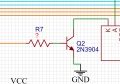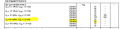# How to calclate Rb and Rc value for NPN transistor?

Thread Starter

#### Beginner0001

Joined Sep 29, 2017
18
Here is a part of my schematic.
K pin from LCD is connected to NPN transistor. A pin is connected to Rc and Vcc, 5V.
Base is connected to uController. It is Atmega16. 5V supply.
I am using 2N3904 transistor as it is showed at the picture.
Electrical Characteristics form datasheet:
Vcbo= 60V
Vceo=40V
Vebo=6V
Ic=200mA
So the question is how can i calculate resistance of Rb(R7 on schematics) and Rc?
I found some calculators online and there's always Vi, explained as the input switching voltage or the input trigger voltage. I am not sure what it refers to.#### ericgibbs

Joined Jan 29, 2010
12,258
hi B,
Is the K and A part of the LCD back light circuit?
E

#### crutschow

Joined Mar 14, 2008
26,771
What control voltage and current does input K expect?

If it's 0 and 5V with low current, then use a nominal 10kΩ from the +5V to Q2's collector.
You want the base current to be about 1/10th to 1/20th of the collector current to fully turn on the transistor into saturation, so make R7 about 100kΩ.

#### Irving

Joined Jan 30, 2016
1,162
I'd imagine so, K & A are standard designations for the backlight LED Kathode and Anode.

The value for Rb is determined by the required collector current Ic in the transistor, which is the current flowing through the backlight LEDs. The current needed into the base terminal of the transistor is the collector current, Ic, divided by the DC current gain of the transistor (sometimes shown as β or hfe) at the current specified.

Lets say you wanted Ic = 50mA, then from the 2N3904 data-sheet the gain at 50mA is around 60 (see snippet below, use the value at or near the required current) so your required base current is approx 50/60 = 0.83mA. The voltage at the uController output will be around 4v and the base voltage of the transistor needs to be 0.7v so Rb = (4 - 0.7)/0.83 kOhm or approx 4k, any value from 2.7k to 3.9k would probably do in this situation. Obviously do the calculation for your own value of Ic; the important thing is you want the transistor to be saturated, or fully turned on, so go for a value of resistor at least one or two steps below the calculated value.You may, or may not need an Rc, it depends on the supply voltage, Vf, the forward voltage of the backlight LEDs and the
value of Vce(sat) - the voltage drop across the transistor at the specified Ic when fully turned on.

For example the backlight on a typical 1602 16x2 LCD has a current of 100mA, and a Vf of 4.1v. Vce(sat) at 100mA of a 2N3904 transistor is about 0.3v. So on a 5v supply, the voltage drop across Rc is 5 - 4.1 - 0.3 = 0.6v. At 100mA that's a resistance of 0.6/0.1 = 6ohms. I'd probably not bother with one, but check the actual value of Ic once built and adjust Rb, if necessary, to control the collector current. A different model OF 1602 has a Vf of 3v and a backlight current of 25mA. In that case voltage over Rc = 5 - 3 - 0.3 = 1.7v at 25mA = 68ohm, i'd definitely put this in.

Last edited:
Thread Starter

#### Beginner0001

Joined Sep 29, 2017
18
hi B,
Is the K and A part of the LCD back light circuit?
E
yes, it is anode and cathode of led.

#### crutschow

Joined Mar 14, 2008
26,771
We can't give you a good answer which such little information.
Do you want to control the intensity of the backlight?
What is the backlight current value (or range of currents)?

Thread Starter

#### Beginner0001

Joined Sep 29, 2017
18
We can't give you a good answer which such little information.
Do you want to control the intensity of the backlight?
What is the backlight current value (or range of currents)?
Yes, that is the goal.
Here are the data from datasheet. It is 16x2 LCD.
Backlight supply voltage: 3V
Backlight supply current: for 3.3V and R=25ohm, I=16mA

#### crutschow

Joined Mar 14, 2008
26,771
Backlight supply current: for 3.3V and R=25ohm, I=16mA
Then for 5V and 16mA, R in series with the transistor collector would be 130 ohms.

The base resistor should be sized to provide about 1/10th of 16mA, or 1.6mA.
Rb is thus (5V-0.7V) / 1.6mA = 2.7kΩ.

To vary the brightness, you could generate a PWM signal from the Atmega output.

•SamR